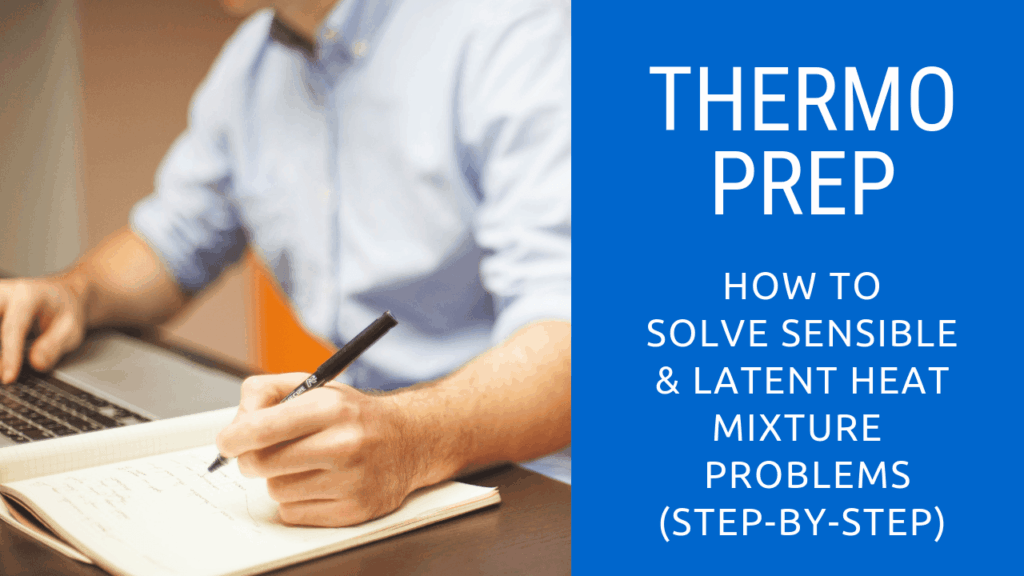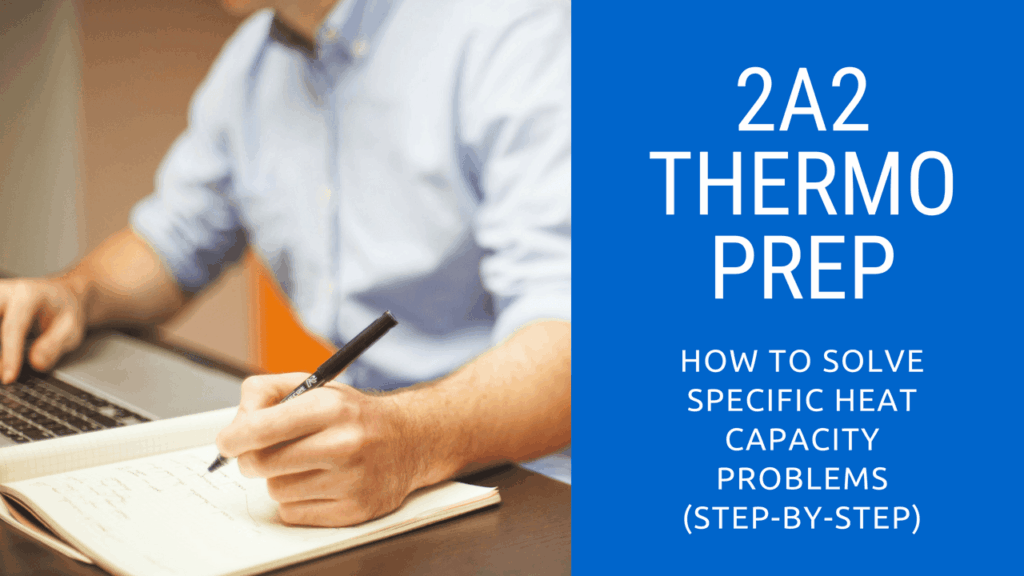# 2A2 Thermodynamics – Heat## How To Solve Sensible and Latent Heat Mixture Problems (Step-By-Step)

Not sure How To Solve Sensible and Latent Heat Mixture Problems? This video will show you step by step on how to solve various sensible and latent heat mixture problems that may show up on your 2A2 exam. A variety of examples and practice problems are included along with a downloadable worksheet (link below). How …## How to Solve Water Equivalent Calculations (2nd Class)

Todays tutorial covers How to Solve Water Equivalent Calculations to prepare you for your Second Class 2A2 exam. The tutorial explains the concept of water equivalent and includes several practice problems in a Free PDF Download of how to derive the formula, determine the water equivalent and how to apply the calculated water equivalent to solve heat …## How to Solve Specific Heat Capacity Calculations (2nd Class)

Todays tutorial covers How to Solve Specific Heat Capacity Calculations to prepare you for your Second Class 2A2 exam. The tutorial explains the concept of specific heat capacity and includes several practice problems including a Free PDF Download of how to use the formula, find the specific heat and how to use specific heat in calculations …